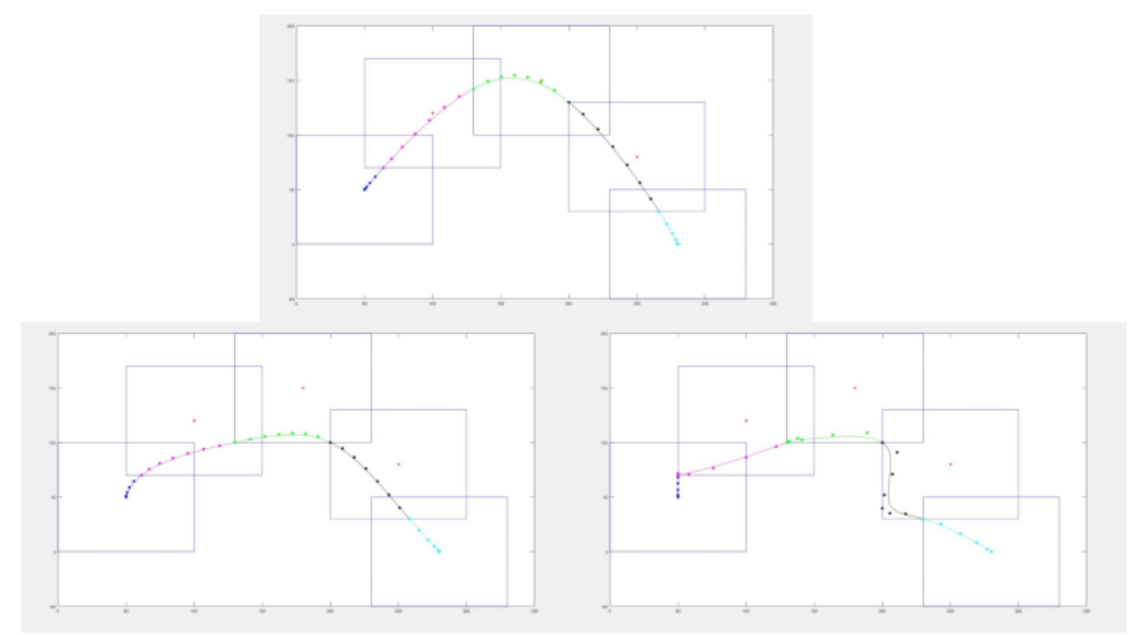• 时间:
• 浏览:

# Introduction

## Minimum snap trajectory optimization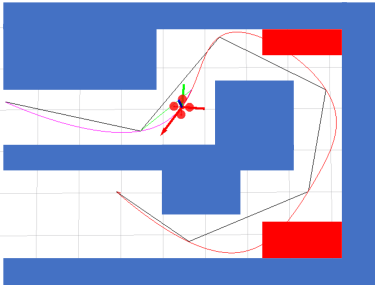•计算成本低，易于实现。
•对轨迹本身没有约束。
•轨迹的“超调”不可避免。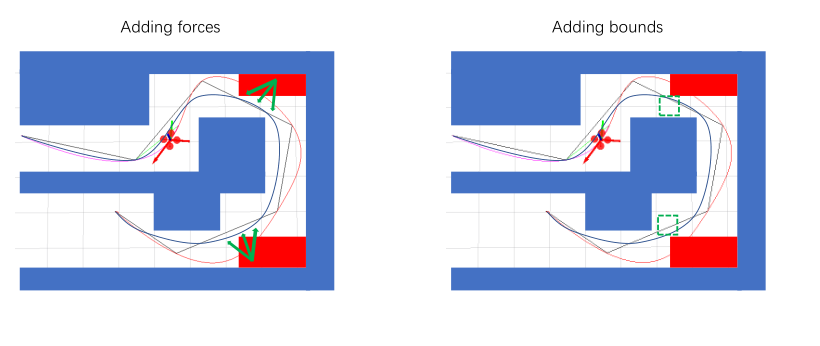## Hard/Soft constraints

min ⁡ f ( x ) s . t . g i ( x ) = c i , i = 1 , . . . , n    等式约束    h j ( x ) ⩾ d j , j = 1 , . . . , n    不等式约束 \min f\left( x \right) \\ s.t. g_i\left( x \right) =c_i, i=1,...,n\,\,\text{等式约束} \\ \,\, h_j\left( x \right) \geqslant d_j, j=1,...,n\,\,\text{不等式约束}

min ⁡ f ( x ) + λ 1 ⋅ g ( x ) + λ 2 ⋅ h ( x ) \min f\left( x \right) +\lambda _1\cdot g\left( x \right) +\lambda _2\cdot h\left( x \right)
λ \lambda 后面惩罚项/损失函数
•首选但不是严格要求的约束。
•包含各种损失函数。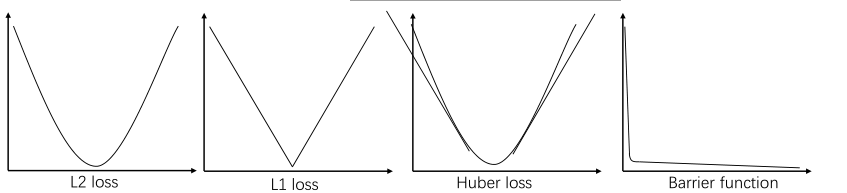# Hard-constrained Optimization

## Corridor-based Trajectory Optimization

[论文](Online generation of collision-free trajectories for quadrotor flight in unknown cluttered environments , Jing Chen et al.)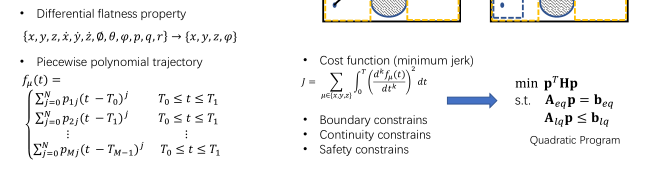### Problem formulation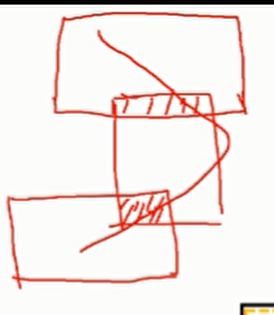• 瞬时线性约束，比较好施加
• 起始点，重点约束（ A p = b A_p=b
• 变化点 （ A p = b ， A p ⩽ b A_p=b，A_p \leqslant b )
• 连续约束（ A p i = A p ( i + 1 ) A_{p_i}=A_{p_(i+1)}
• 间隔线性的约束
• 对于 A ( t ) p ⩽ b , ∀ t ∈ [ t l , t r ] A\left( t \right) p \leqslant b, \forall t\in \left[t_l,t_r \right]
• 边界约束
• 动力学约束（v,a限制)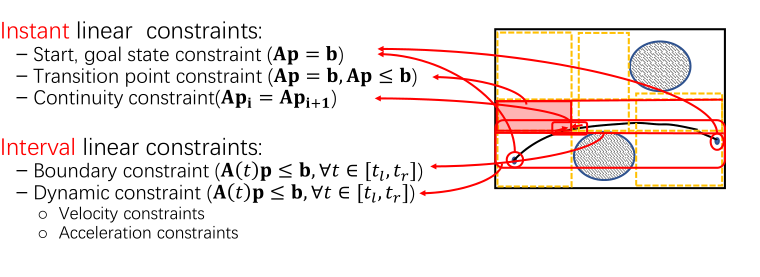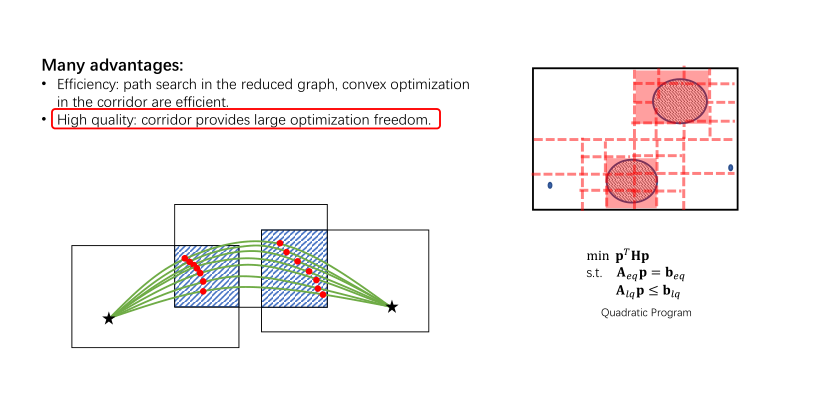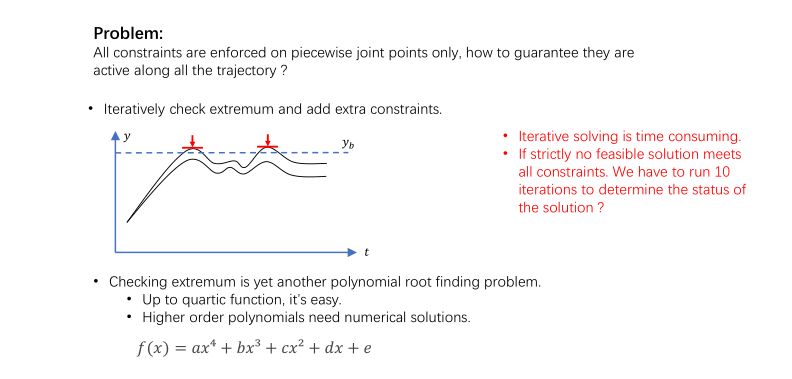• 但是QP问题迭代求解非常耗时。
• 如果没有严格可行的解决方案满足所有约束。那我们要跑10次迭代来确定解决方案?
• 原因:
•检验极值(多项式求极值转变为导数为0的问题)是另一个多项式求根问题。
•四次等式有求根公式，容易求,但是高阶呢。

### Polynomial roots finding

•高阶多项式需要数值解，使用伴随矩阵，转化为矩阵求根问题.在matlab的root公式中说明如下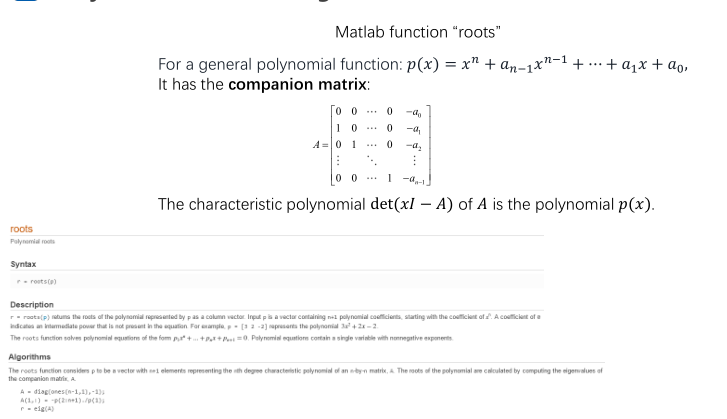## Bezier Curve Optimization贝塞尔曲线优化

Y:替换多项式的形式,因为其不方便施加约束

### Trajectory basis changing

•将轨迹的基从单项多项式改为伯恩斯坦多项式
•Bézier曲线只是一个特殊的多项式，它可以通过以下方法映射为单项多项式:
p = M c ˙ p=M \dot c 之前的推导仍然成立。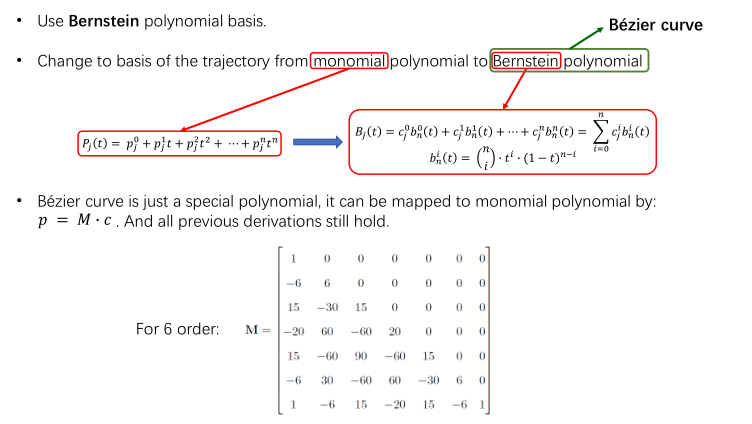[ n i ] 相当于 c n i \left[ \begin{array}{c} n\\ i\\ \end{array} \right] \text{相当于}c_{n}^{i}

Properties:
Bezier的系数c称为控制点，有实际的物理意义
•端点插值。贝塞尔曲线总是从第一个控制点开始，到最后一个控制点结束,绝不通过任何其他控制点。如图
•凸包。贝塞尔曲线𝐶(𝑢)由一组完全受限的控制点ci组成,一段Bezier曲线一定被其所有控制点围成的凸包包围住(P0~P4)
•Hodograph。Bezier曲线B(𝑢)的导数曲线B’(𝑢)被称为Hodograph，还是贝塞尔曲线，

• 固定的时间间隔。Bezier曲线总是在[0,1]上定义,所以需要轨迹进行缩放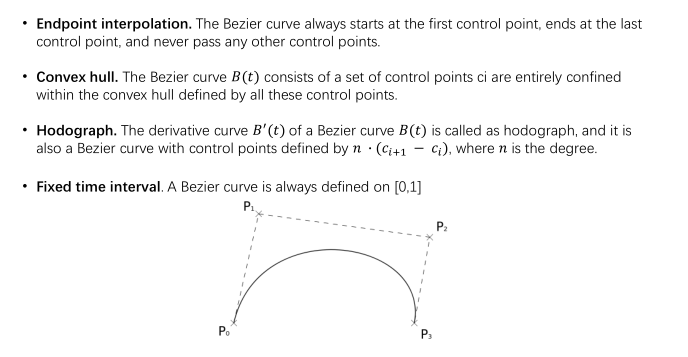### Convex hull property

•飞行走廊由凸多边形组成。
•每一个立方体对应着一条贝塞尔曲线。
•这条曲线的控制点被约束在多边形内
•轨迹完全在所有点的凸包内### Trajectory Generation Formulation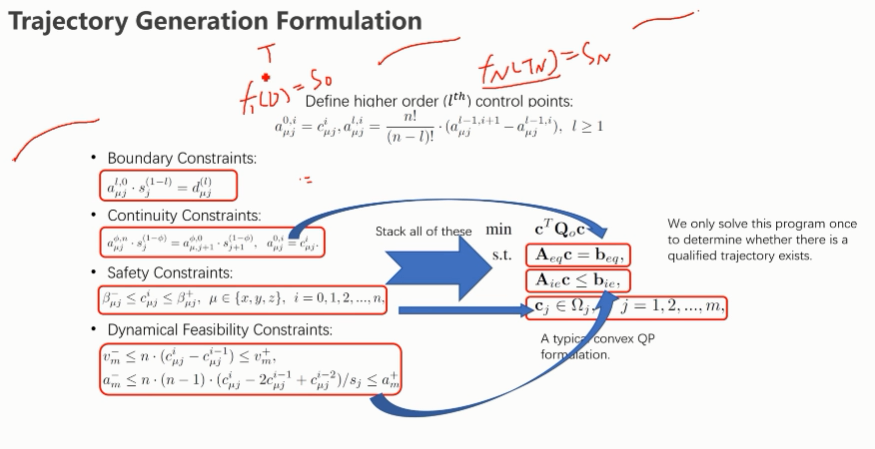## Simulation result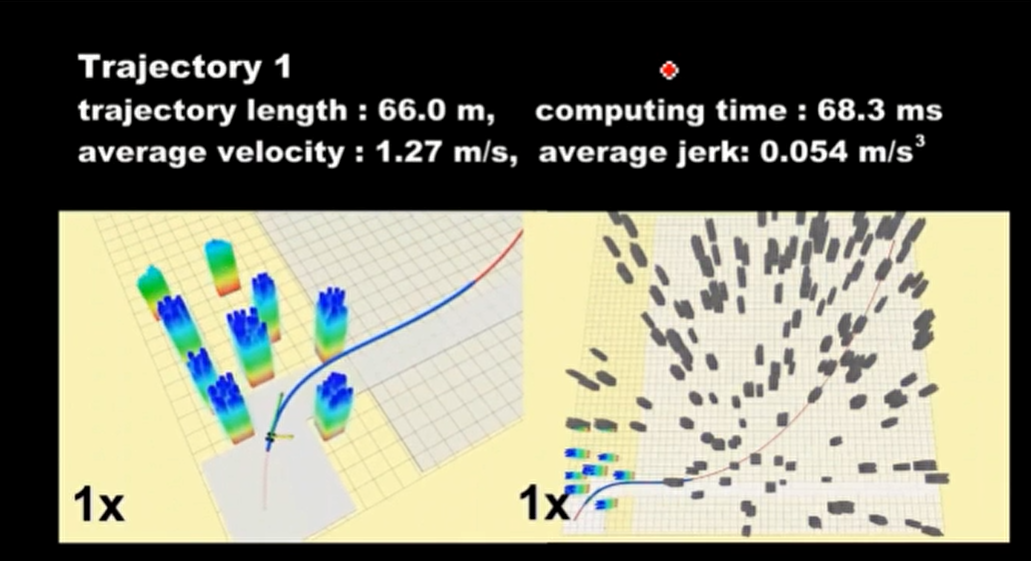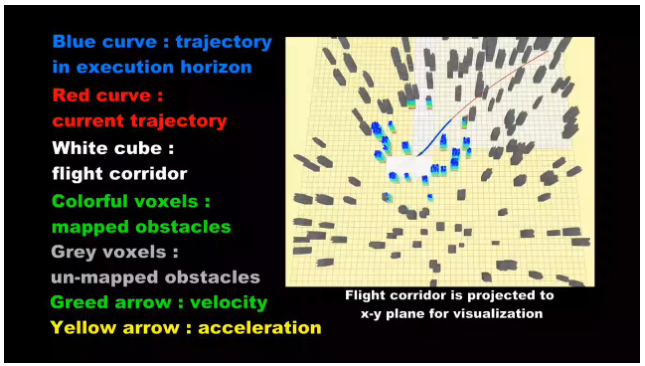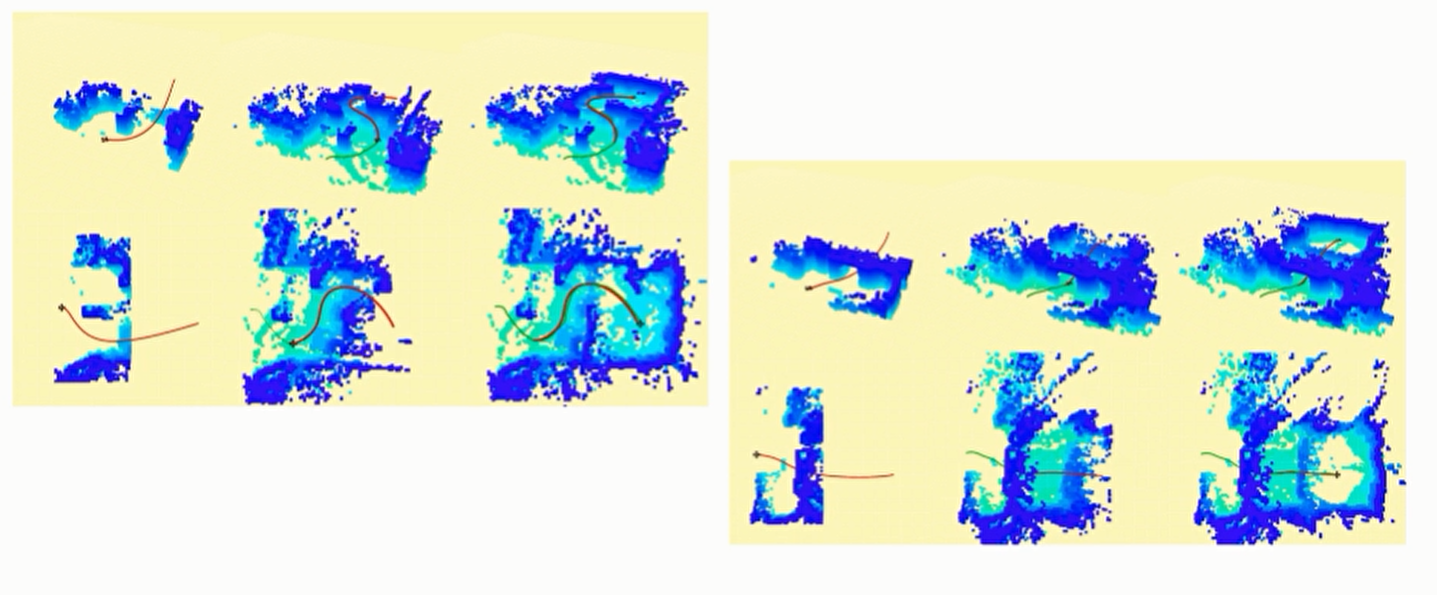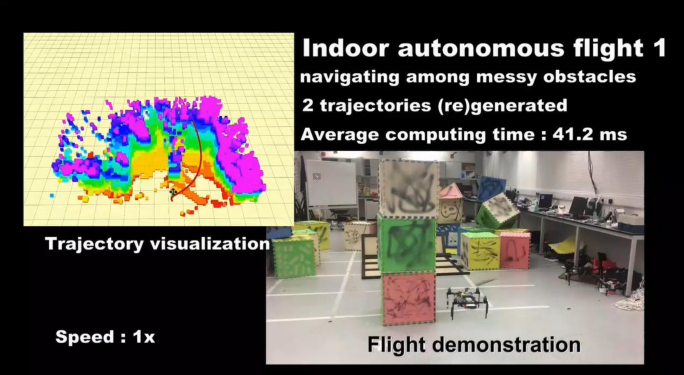[论文](Online Safe Trajectory Generation For Quadrotors Using Fast Marching Method and Bernstein Basis Polynomial , Fei Gao et al.)
source code

## Other Options

### Dense constraints

•在离散时间点添加大量约束。
•每一个时间段恒定加速度。
•QP方案解决方案

• 速度慢，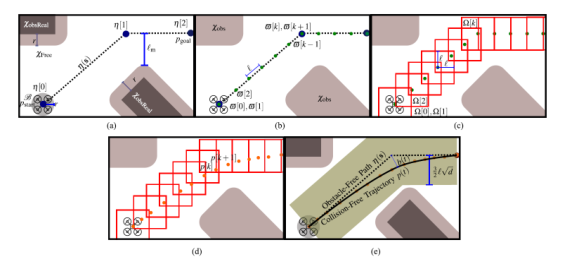•总是生成过于保守的轨迹。
•约束太多，计算负担高

[论文](A hybrid method for online trajectory planning of mobile robots in cluttered environments , L Campos-Macías et. al)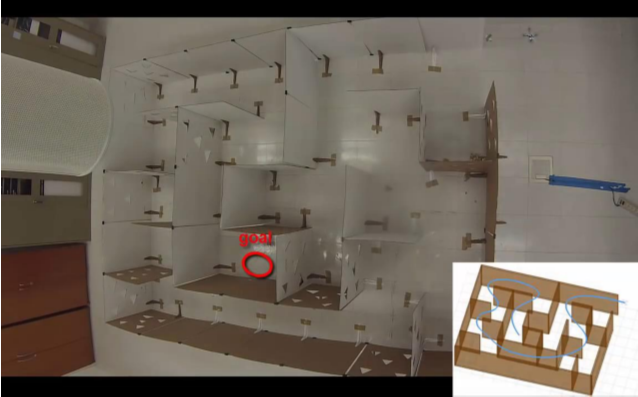### Mixed integer optimization

[论文](Mixed-integer quadratic program trajectory generation for heterogeneous quadrotor teams , D. Mellinger et al.)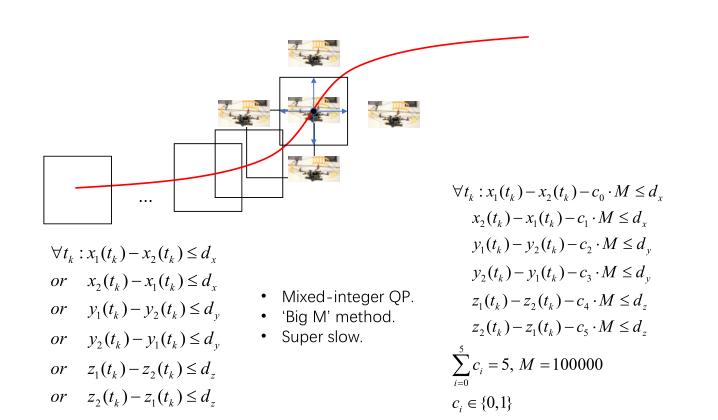# Soft-constrained Optimization

## Distance-based Trajectory Optimization

• Motivation

Vison-based无人机:
•有限的传感范围和质量
•噪声深度估计
Hard-constrained方法:
•所有解空间都是等价的
•解空间对噪音敏感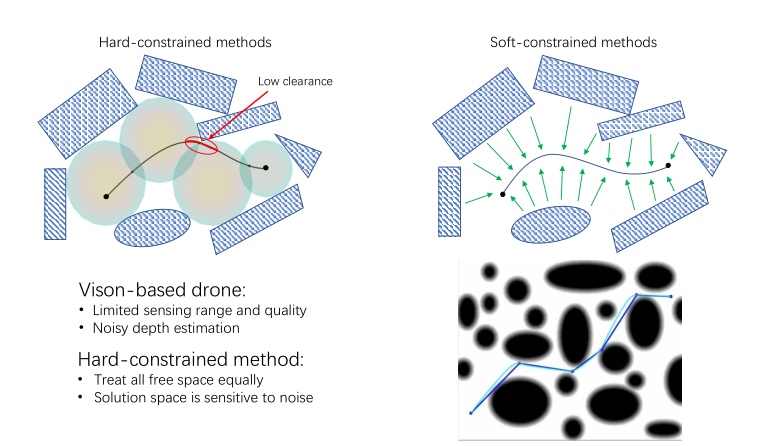### Problem formulation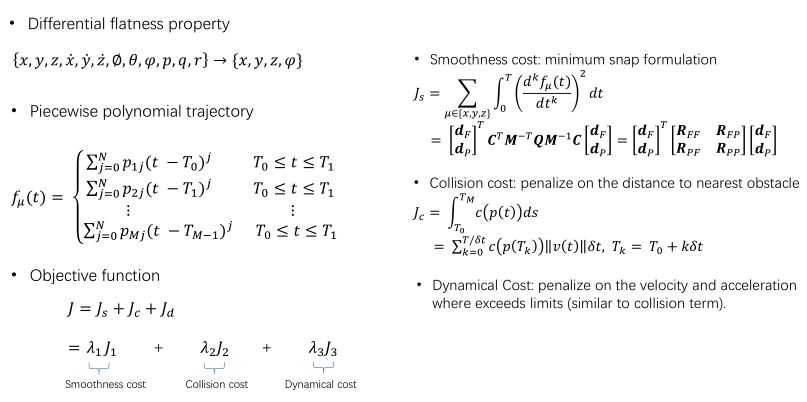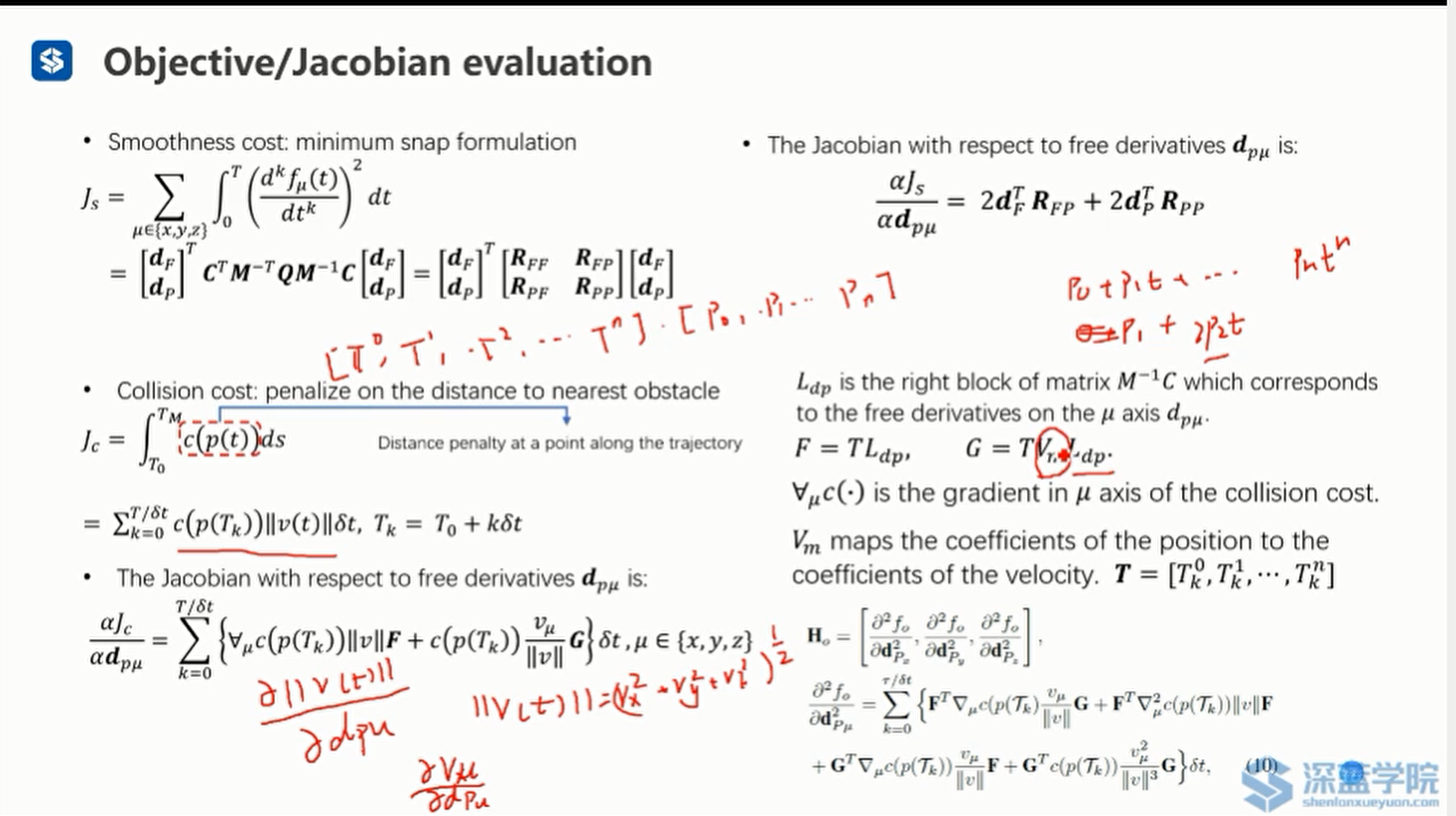• 利用微分平坦性将无人机参数空间降维，并用多项式模拟轨迹
• 定义目标函数,第一项为平滑项、第二项为碰撞项、第三项为动力学约束项。
• 平滑项可以使用Minimum Snap求解公式求解,用中继点的(p,v,a)来代替路径多项式的参数,但在这里中继点的位置是可以改变的
• 碰撞项,惩罚距离最近的障碍物的轨迹点(对曲线沿着路径进行积分ds),然后分解为小段之和. p ( t ) p(t) 表示t时刻位置, c ( p ( t ) ) c(p(t)) 为惩罚函数,涉及到距离场;二者的确定方式:首先可以构建一个Euclidean signed distance field（ESDF），每个格子储存其到障碍物的最小距离。然后再经过一个幂次函数，或者barrier function，使得当里障碍物特别近的时候，惩罚急剧上升。
• 由于是对路径积分，求导不太好求，所以把路径离散化掉再对 d p u d_{pu} 求导, c ( p ( T k ) ) 和 ∣ v ( t ) c(p(T_k)){和}|v(t) 与其有关, δ t \delta t 是常量
• 动力学项,惩罚速度和加速度,与碰撞项类似,不过 c v c_v 不用构建ESDF.
• 目标函数(ESDF,这是由于距离场的特性决定的)并非凸函数,不能直接凸优化求解,而需要通过一步步求导,平滑项 J s J_s ,碰撞项对自由变量 d p u d_{pu} 求导,得到关于它的雅可比矩阵

### Euclidean signed distance field (ESDF)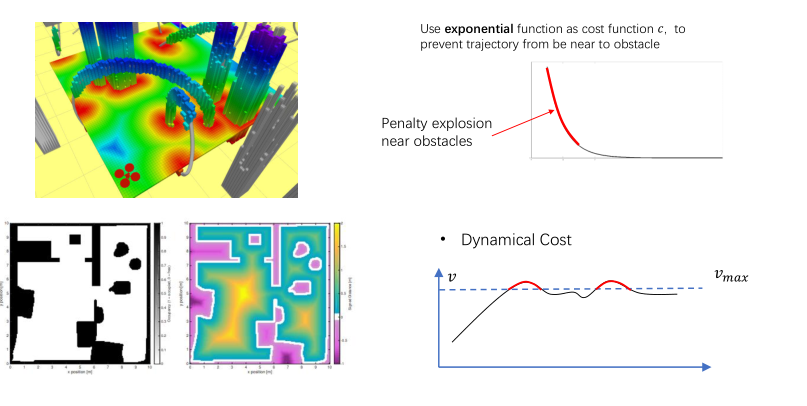### Numerical optimization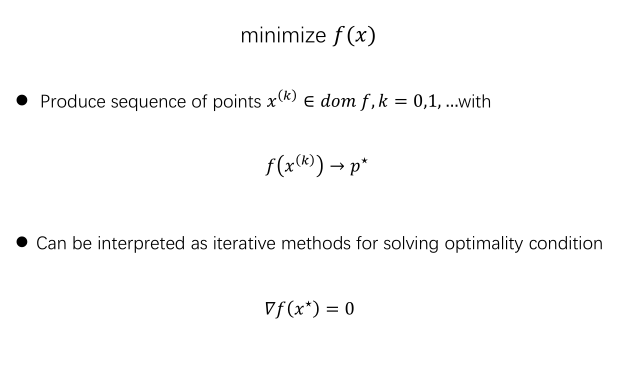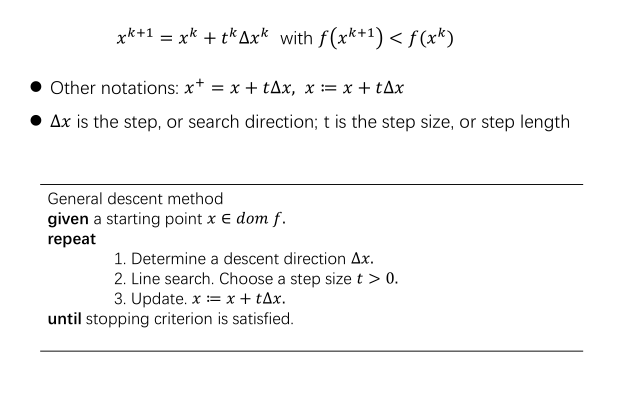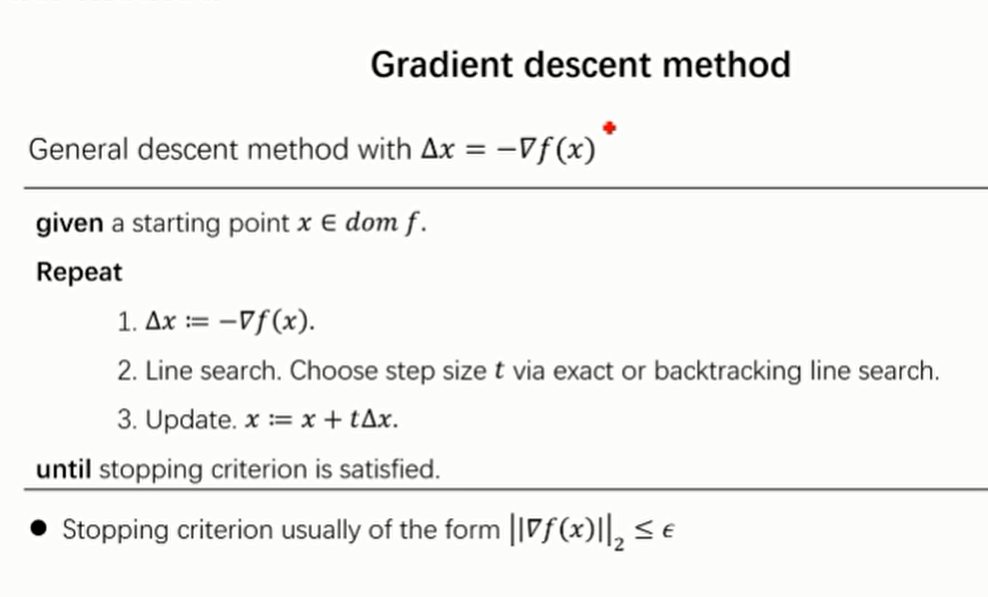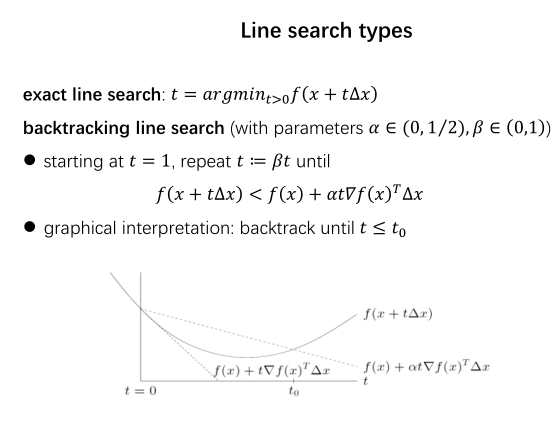• 二阶方法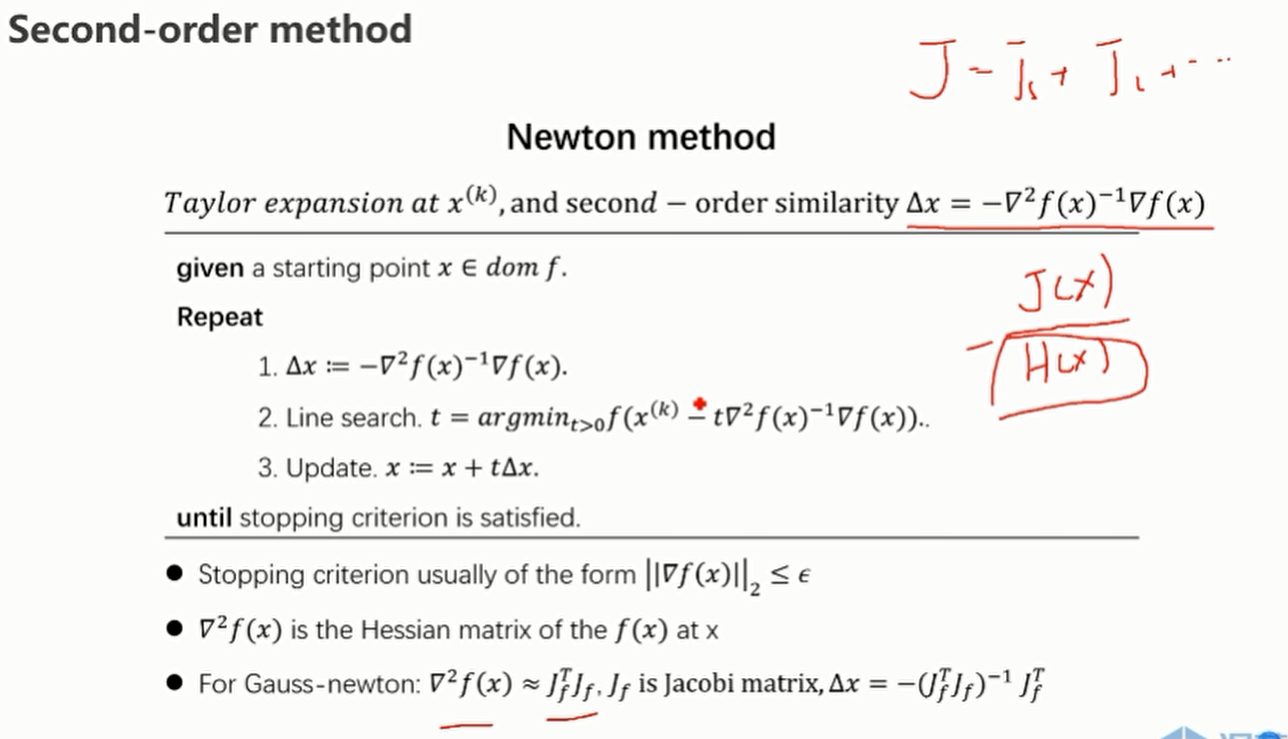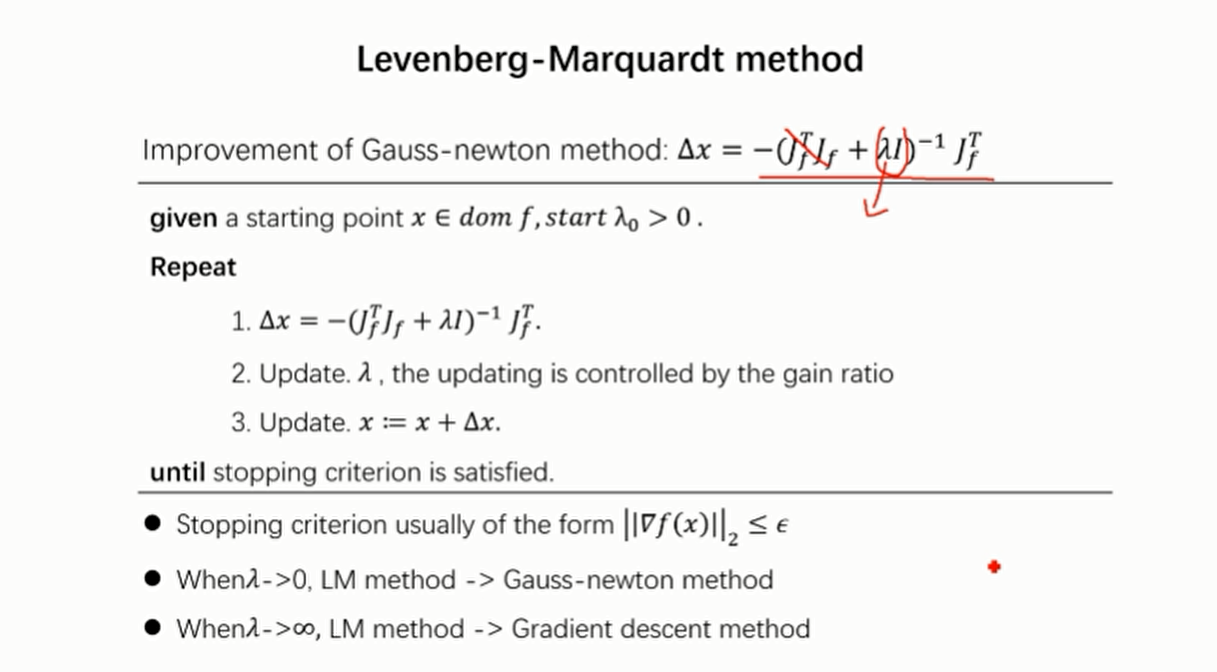### Planning strategy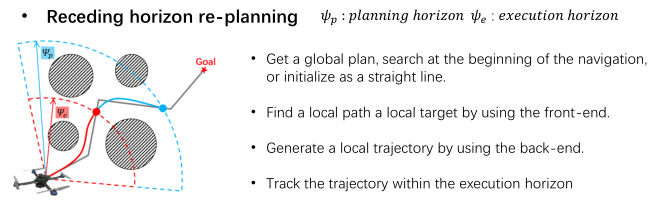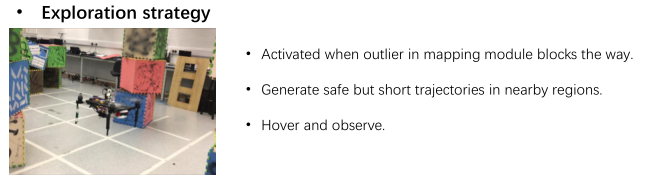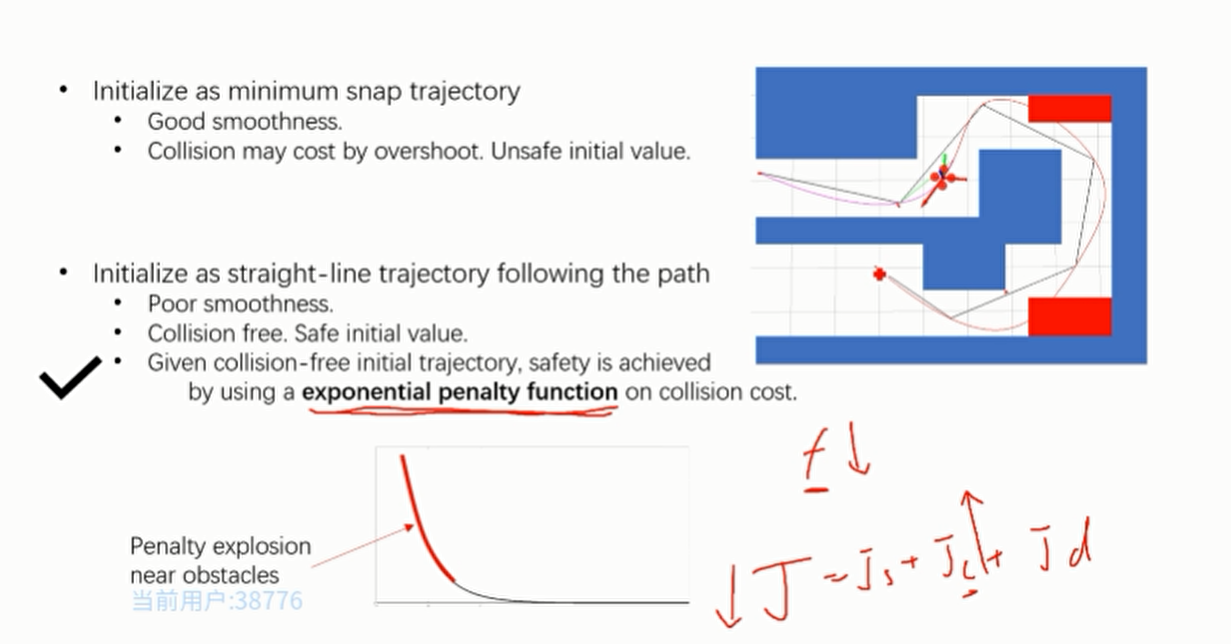### optimization strategy

optimize
optimal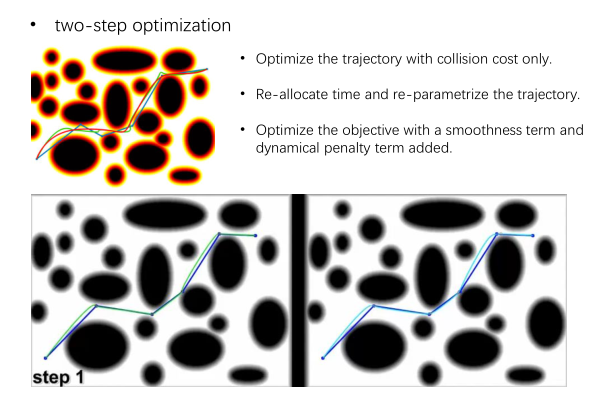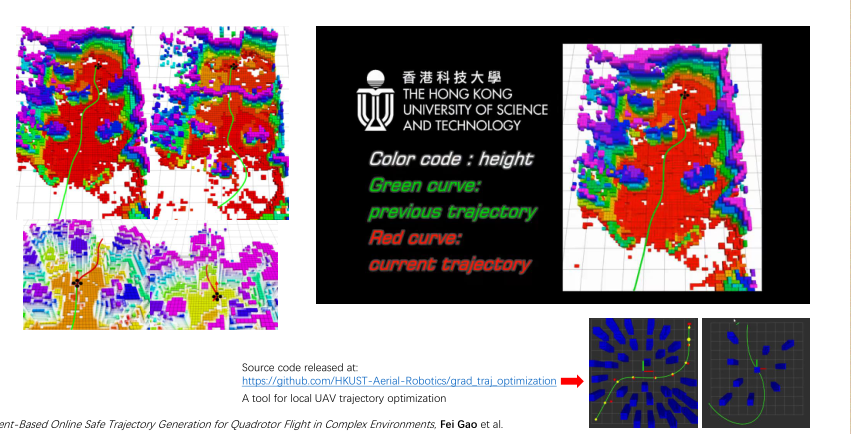Source code

# Case Study

## Fast planner

Kinodynamic path searching

• B-Spline trajectory optimization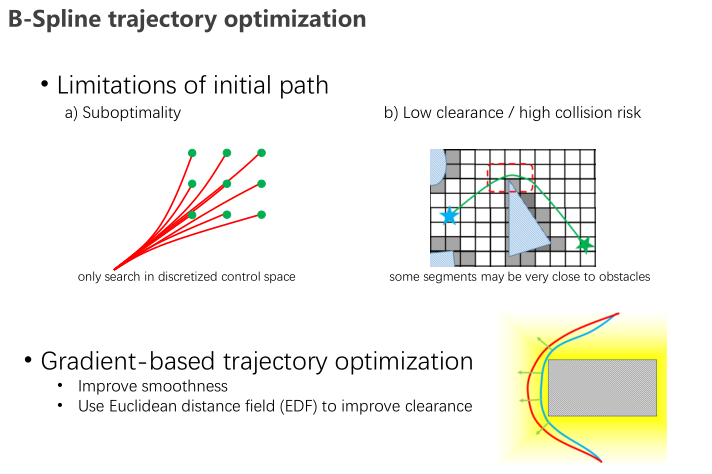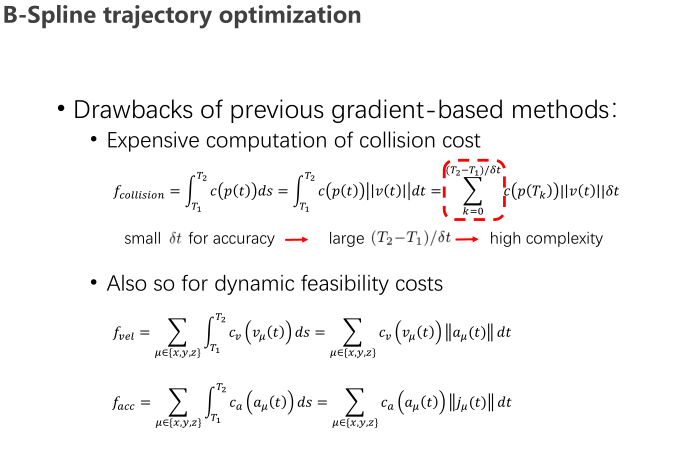B样条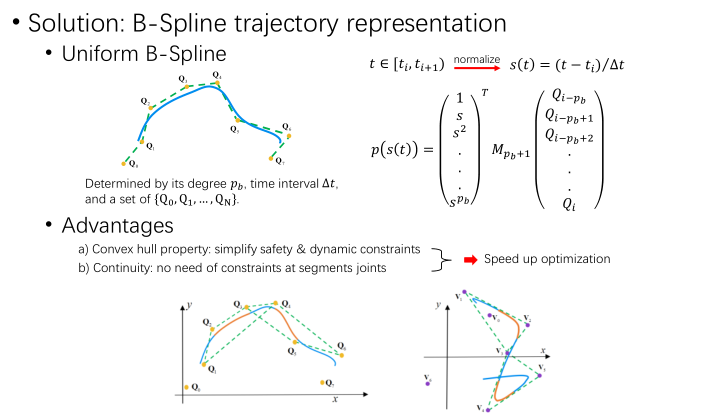优化速度的提升
凸包特性:简化安全与动力约束
连续性:节段连接处不需要约束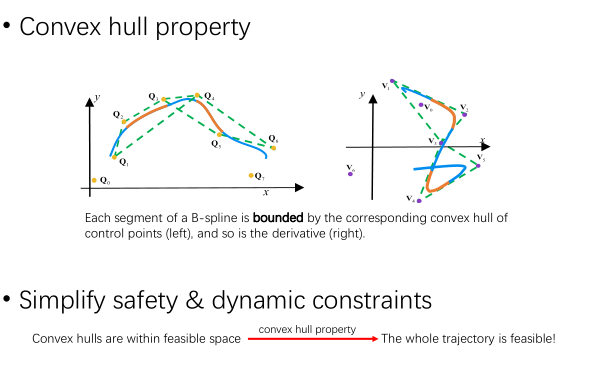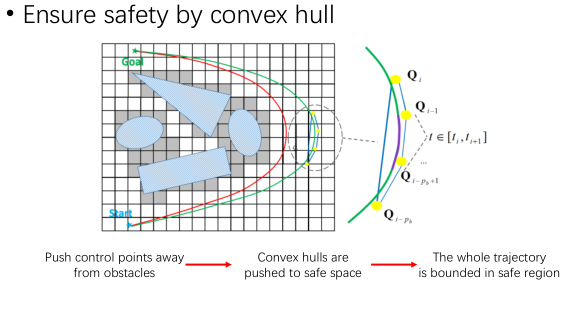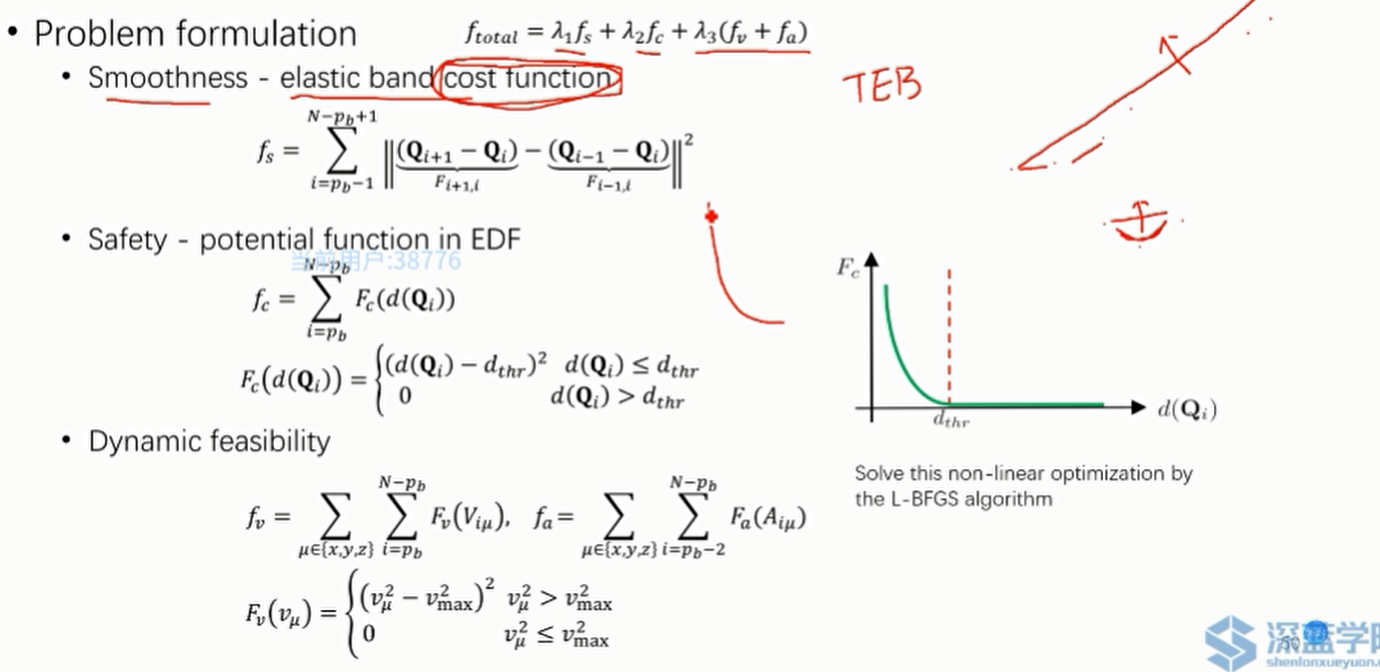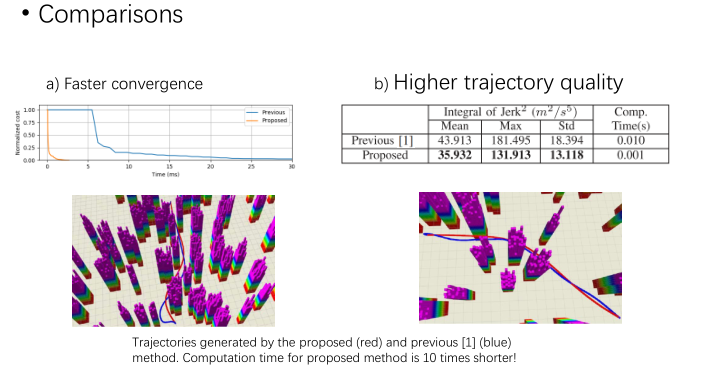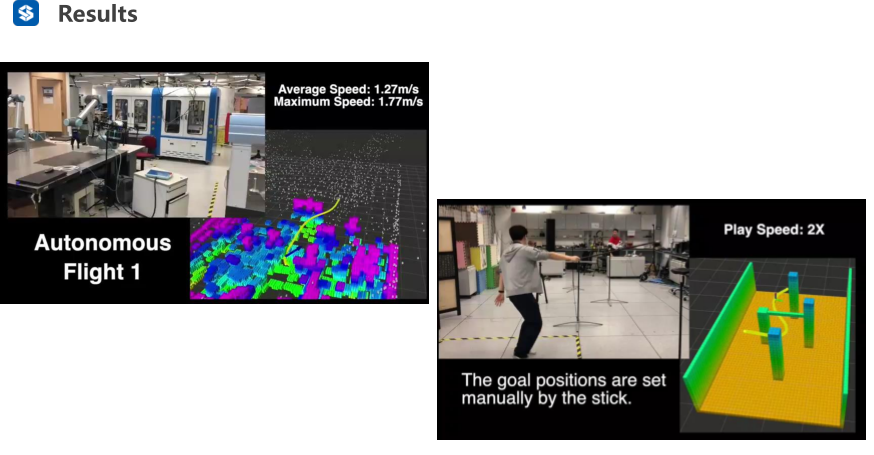# Homework

•在matlab中编写一个生成走廊约束分段贝塞尔曲线。
•给出了贝塞尔到单项多项式的转换。
•走廊是预先设定好的。
•只需要限制位置。